# NCERT Solutions class-11 Chemistry Thermodynamics## myCBSEguide App

Complete Guide for CBSE Students

NCERT Solutions, NCERT Exemplars, Revison Notes, Free Videos, CBSE Papers, MCQ Tests & more.

THERMODYNAMICS
1. Choose the correct answer. A thermodynamic state function is a quantity

(i) used to determine heat changes

(ii) whose value is independent of path

(iii) used to determine pressure volume work

(iv) whose value depends on temperature only.

2. For the process to occur under adiabatic conditions, the correct condition is:

(i) ΔT = 0

(ii) Δp = 0

(iii) q = 0

(iv) w= 0

3. The enthalpies of all elements in their standard states are:

(i) unity

(ii) zero

(iii) < 0

(iv) different for each element

4. ΔU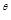¸of combustion of methane is – XkJ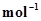. The value of ΔH¸is

(i) = ΔU(ii) > ΔU(iii) < ΔU(iv) = 0

5. The enthalpy of combustion of methane, graphite and dihydrogen at 298 K are, -890.3 kJ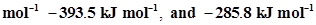respectively. Enthalpy of formation of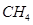(g) will be

(i) –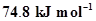(ii)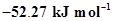(iii)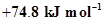(iv)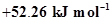.

6. A reaction, A + B → C + D + q is found to have a positive entropy change. The reaction will be

(i) possible at high temperature

(ii) possible only at low temperature

(iii) not possible at any temperature

(iv) possible at any temperature

7. In a process, 701 J of heat is absorbed by a system and 394 J ofwork is done by the system. What is the change in internal energy for the process?

8. The reaction of cyanamide,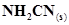,with dioxygen was carried out in a bomb calorimeter, and ΔU was found to be -742.7 kJat 298 K. Calculate enthalpy change for the reaction at 298 K.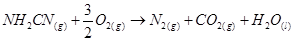9. Calculate the number of kJ of heat necessary to raise the temperatureof 60.0 g of aluminium from 35°C to 55°C. Molar heat capacity of Al is 24 J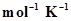.

10. Calculate the enthalpy change on freezing of 1.0 mol of water at 10.0°C to ice at -10.0°C.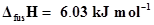at 0°C.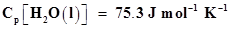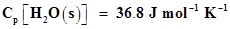11. Enthalpy of combustion of carbon to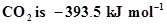. Calculate the heat released upon formation of 35.2 g of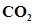from carbon and dioxygen gas.

12. Enthalpies of formation of CO(g),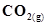,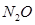(g) andare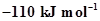,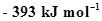,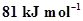and 9.7 kJrespectively. Find the value of Δ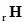for the reaction: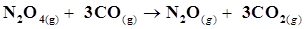13. Given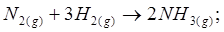;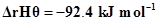What is the standard enthalpy of formation of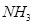gas?

14. Calculate the standard enthalpy of formation of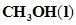) from the following data: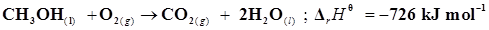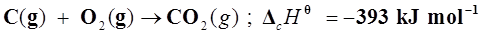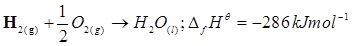15. Calculate the enthalpy change for the process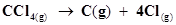and calculate bond enthalpy of C-Cl in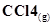.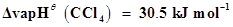.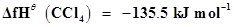.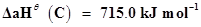, where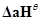is enthalpy of atomisation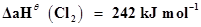16. For an isolated system, ΔU = 0, what will be ΔS?

17. For the reaction at 298 K,

2A + B → C

ΔH = 400 kJand ΔS = 0.2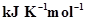At what temperature will the reaction become spontaneous considering ΔH and ΔS to be constant over the temperature range?

18. For the reaction,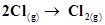,what are the signs of ΔH and ΔS ?

19. For the reaction 2A(g) + B(g) → 2D(g) . Calculate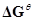for the reaction, and predict whether the reaction may occur spontaneously.

20. The equilibrium constant for a reaction is 10. What will be the value of? R = 8.314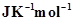, T= 300 K.

21. Comment on the thermodynamic stability of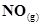, given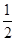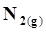+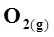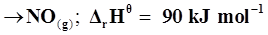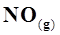+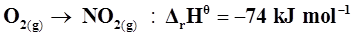22. Calculate the entropy change in surroundings when 1.00 mol of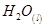is formed under standard conditions.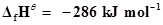.## Test Generator

Create Papers with your Name & Logo